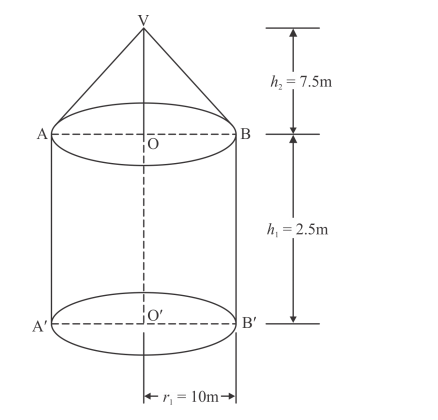# A tent is in the form of a cylinder of diameter 20 m and height 2.5 m,

Question:

A tent is in the form of a cylinder of diameter 20 m and height 2.5 m, surmounted by a cone of equal base and height 7.5 m. Find the capacity of the tent and the cost of the canvas at Rs 100 per square metre.

Solution:

Given that:

Radius of the base $r=\frac{d}{2}=\frac{20}{2}=10 \mathrm{~m}$

Height of the cylinder $h_{1}=2.5 \mathrm{~m}$

Height of the cone $h_{2}=7.5 \mathrm{~m}$Slant height of the cone

$l=\sqrt{r^{2}+h^{2}}$

$=\sqrt{10^{2}+7.5^{2}}$

$=12.5 \mathrm{~m}$

The total capacity of the tent is given by

$V=\pi r^{2} h_{1}+\frac{1}{3} \pi r^{2} h_{2}$

$=\pi \times 10^{2} \times 2.5+\frac{1}{3} \times \pi \times 10^{2} \times 7.5$

$=\pi \times 250+\pi \times 250$

$=500 \pi \mathrm{m}^{3}$

The total area of canvas required for the tent is

$S=2 \pi r h_{1}+\pi r l$

$=2 \times 3.14 \times 10 \times 2.5+3.14 \times 10 \times 12.5$

$=\pi(2 \times 10 \times 2.5+10 \times 12.5)$

$=\pi(50+125)$

$=\frac{22}{7} \times 175$

$=550 \mathrm{~m}^{2}$

Therefore, the total cost of the canvas is

$=100 \times 550$

$=$ Rs. 55000

Hence, the total capacity and cost is $V=500 \pi \mathrm{m}^{3}$, and Rs. 55000